# Photocoupler-Free Isolated Flyback DC-DC ConverterMethod for Regulating the Secondary by Controlling the Primary

2017.06.22

－Just looking at the circuit diagram, it looks like an unregulated isolated power supply; is the output really regulated?

Of course. Allow me to explain how the output of the BD7F series is regulated. The explanation will be easier using graphics, which I have prepared.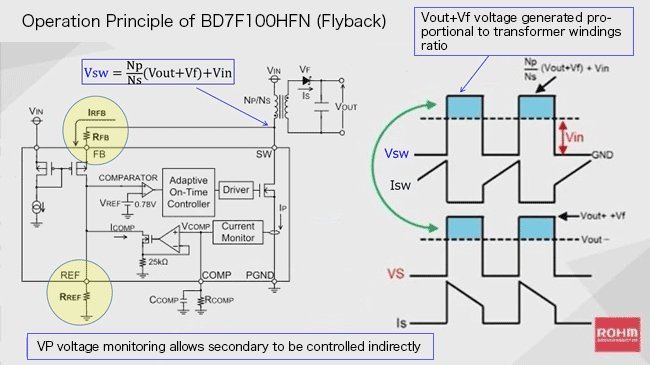The circuit diagram on the left side of the graphic shows the simplified circuit block for the DB7F series device, an external transformer, and a secondary-side rectifying circuit. On the right side are waveforms of the voltage (Vx) and current (Ix) at each node.

How to perform regulation without feedback from the secondary side? The flyback voltage VSW on the primary side contains Vout as a component. Then VSW is monitored and operation is performed to indirectly detect Vout for regulation control.

In order to explain in a little more detail, I’ll ask you to look at the waveforms and the equation within the graphic. Vsw is the primary-side flyback voltage, and is generated, when the internal switch (MOSFET) turns off, at the point at which one end of the transformer primary-side windings is connected to the SW pin of this IC. This VSW is the result of the product of the “transformer windings ratio (primary-side Np/secondary-side Ns)” and the “sum of Vout and the output rectifying diode voltage Vf”, to which Vin is added. Put simply, the voltage is the result of adding Vin to the voltage (vout+Vf) multiplied by the windings ratio of the transformer. But rather than an explanation, the waveforms and equation are easier to understand. One thing must be noted though: this equation has been simplified to make it easier to grasp. The voltage appearing on the secondary side is represented at (Vout+Vf), but strictly speaking, it is necessary to add a voltage resulting from the total secondary-side impedance (the windings resistance and ESR), multiplied by IS. This is an error factor, and in actual design the term must be included in calculations.

－I see that the primary-side flyback voltage is used. I understand that it is used in some kind of operation, but if it’s not too complicated, could you explain just how this works?

The basic principle is not all that difficult. But, it is easier to understand using a circuit diagram and an equation, and so let’s have a look at another diagram and another equation. What’s important here are the two places that are circled in the circuit diagram. Details are indicated in the product data sheet, and the equation from the data sheet is not the simplified version we presented above, but includes all the terms for the secondary-side voltage, and so for consistency, from here on we will use the data sheet equation. The symbol notation (lowercase, subscripts) are somewhat different, but the meanings are the same.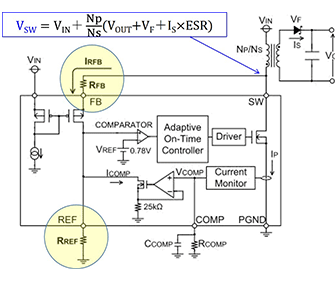The following is the basic equation. VSW is the flyback voltage at the SW pin. Here “IS×ESR” is added to the previous simplified equation.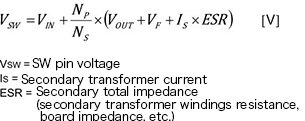VSW is converted into the current IRFB by the resistor RFB. The voltage VFB at the FB pin is roughly equal to the voltage VIN due to the differential circuit with VIN. This operation should be thought of and remembered as like that of the differential circuit of an op-amp, based on loop control. IRFB is represented by the following equation.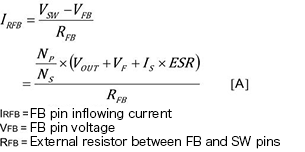VFB≒VIN, and therefore it is clear that the voltage obtained by subtracting VIN (VFB) from VSW, divided by RFB, gives us IRFB. Here, a term with VOUT appears in the equation. From here we shall determine VOUT.

Because this current IRFB flows through the resistor RREF, the voltage VREF at the REF pin is as given by the following equation.

We have already expanded IRFB, and as is only to be expected, retracing gives us VREF＝ RREF × IRFB.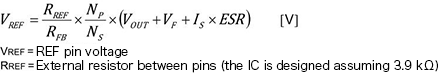Here, let’s look at the circuit diagram. The REF pin voltage is input to the non-inverting pin of the comparator within the IC and is compared with the reference voltage of 0.78 V at the inverting pin. For control ICs in general, this would be an error amplifier, but in this IC the control method uses the comparator, and so the action is that of a comparator. Similarly, to an error amplifier, the voltage at the REF pin (the comparator inverting input) is equal to the reference voltage (0.78 V). Hence the output voltage VOUT and the voltage VREF at the REF pin are related as follows.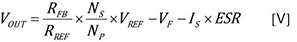In other words, the output voltage VOUT is determined by the transformer windings ratio and the ratio of the resistances RFB and RREF. VF and IS×ESR are factors resulting in output voltage errors.

－I see. I now understand the meaning of using loop control of the primary-side flyback voltage to regulate the output voltage indirectly. During design, then, the output voltage should be set based on this equation?

That is not a problem. The data sheet indicates 3.9 kΩ as the standard value for RREF, and VREF is 0.78 V (typ), and so the output voltage can be set using these values. Moreover, application circuit examples and recommended transformers are provided. By all means, please have a look at the data sheet.

－You have clarified the reasons why a photocoupler or auxiliary windings are unnecessary. Next, I would like to ask about the functions and features of the DB7F series of ICs.

(to be continued）